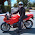## Friday, September 16, 2011

### Statistic analysis using gnuplot (1)

Last time we dealt with the maximum, minimum and mean value of a statistic analysis problem. Today the standard deviation will be added.
The standard deviation is defined as the square root of the variance. With variance stand for the mean squared value minus the squared mean value. The mean value have been dealt last time. We just need to store it. Strange it is, although there is only one data point after the smooth, when I plot it to a data file using the table mode, I get two output points with the second one marked with "u" which means undefined value.(who can tell me why?) In a similar way we plot the mean of squared value to another data file. Next we read this two value out from the data file. And use them to calculate the standard deviation. Since there is only two data points in the file, we use f(x)=ax+b to fit the data, and the fitted result will be exact, then we can use f(x) to get these exact values. At last we plot the standard deviation on the graph. The following is our final plotting script.
```reset
plot "rand_t.dat" u 1:2	#To get the max and min value
ymax=GPVAL_DATA_Y_MAX
ymin=GPVAL_DATA_Y_MIN
ylen=ymax-ymin
xmax=GPVAL_DATA_X_MAX
xmin=GPVAL_DATA_X_MIN
xlen=xmax-xmin
set table "mean.txt"	#put the mean value out
plot "rand_t.dat" u ((xmax+xmin)/2.0):(\$2) smooth unique w p
unset table
set table "mean_squared.txt"	#put the mean of squared value out
plot "rand_t.dat" u ((xmax+xmin)/2.0):(\$2**2) smooth unique w p
unset table
f(x)=a*x+b	#The fit functions
g(x)=c*x+d
fit f(x) "mean.txt" u 0:2 via a,b	#Read the mean and mean of squared value
fit g(x) "mean_squared.txt" u 0:2 via c,d
mean=f(0)	#mean value
mean_squared=g(0)	#mean of the squared value
standard_deviation=sqrt(mean_squared-mean**2)	#standard deviation
print "The mean value is ",mean		#print the mean and standard deviation
print "The standard deviation is ",standard_deviation
#plot
set term post eps enhanced color lw 1.5 font ",20"
set output "statistic.eps"
set xrange [xmin:xmax]
set yrange [ymin-0.5*ylen:ymax+0.5*ylen]
set xlabel "time"
set ylabel "Random Signal"
#The labels
set label 1 at (xmin+xmax)/2.,ymax "Maximum" offset 0,0.5
set label 2 at (xmin+xmax)/2.,ymin "Minimum" offset 0,-0.5
set label 3 at (xmin+xmax)/2.,mean "Mean" offset 0,0.5
set label 4 at (xmin+xmax)/2.,mean+3*standard_deviation \
"Mean+3{/Symbol \163}" offset 0,0.5
set label 5 at (xmin+xmax)/2.,mean-3*standard_deviation \
"Mean-3{/Symbol \163}" offset 0,-0.5
plot "rand_t.dat" u 1:2 w p pt 7 ps 0.5 notitle,\
mean w l lt 2 notitle "Mean",\
ymax w l lt 3 notitle "Maximum",\
ymin w l lt 3 notitle,\
mean+3*standard_deviation w l lt 4 notitle,\
mean-3*standard_deviation w l lt 4 notitle
#the six plot stand for raw data, mean, maximum, minimum,
#3-sigma upper line and 3-sigma lower line
```
At last we get figure like follows.Statistic analysis using gnuplot

1.exellent!

2.Boom. http://www.manpagez.com/info/gnuplot/gnuplot-4.6.0/gnuplot_419.php

1.thanks! works perfectly

3.If I try to run your program, it says:

line 3: undefined variable: GPVAL_DATA_Y_MAX

So where did you define these variables ?